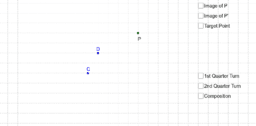•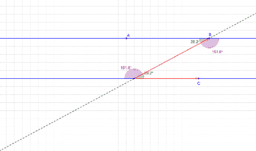Alternate Interior Angles

Activity

•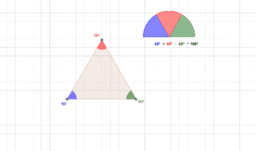Sum of Angles of Triangle

Activity

•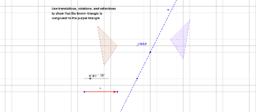Triangle Congruence

Activity

•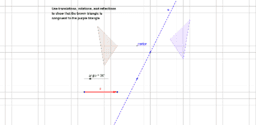Triangle Congruence

Activity

•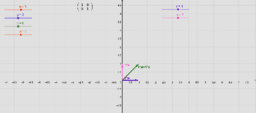Column Space of Plane Transformation

Activity

•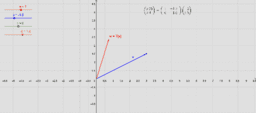Generalized Plane Transformation

Activity

•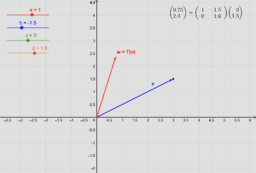Generalized Plane Transformation

Activity

•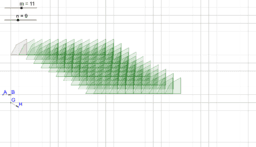Period 6 Translation Animation

Activity

•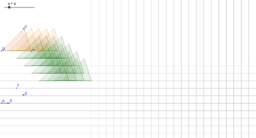Period 2 Translation Animation

Activity

•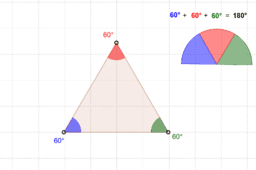Sum of Angles of Triangle

Activity

•Geometry 14-15 Investigations

Book

•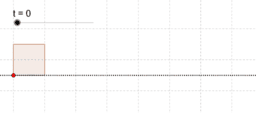Rolling Square

Activity

•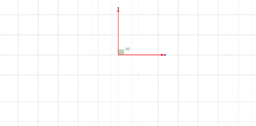Euclidean Geometry

Book

•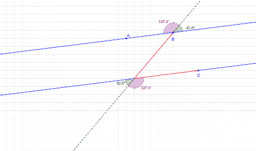Alternate Exterior Angles

Activity

•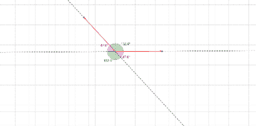Vertical Angles

Activity

•Size of Angle

Activity

•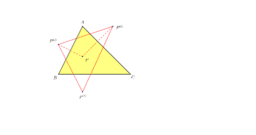Reflections in Triangle Geometry

Book

•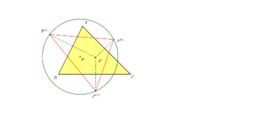Circumcenter of Reflection Triangle

Activity

•Reflection Triangle

Activity

•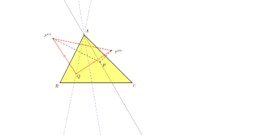Isogonal Line is Perp Bisector Reflection Triangle

Activity

•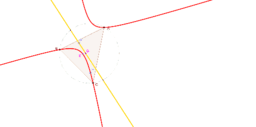Isogonal Conjugate of line through Centroid

Activity

•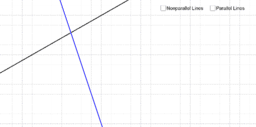Lines and Transversals

Activity

•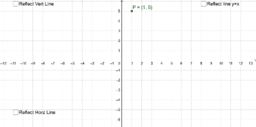Basic Reflections

Activity

•